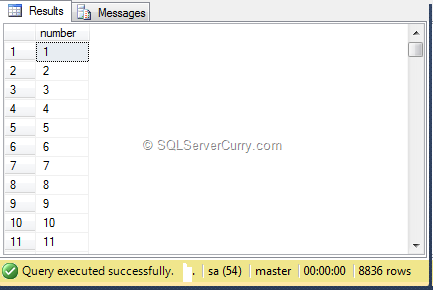### Different Methods To Simulate Cross Join

A Cross join can be effectively used to produce larger dataset that can be used for various purposes. One usage is to generate number table using a CROSS JOIN.

The following code generate series of numbers starting from 1

select
row_number() over (order by s1.name) as number
from
sys.objects as s1 cross join sys.objects as s2

The resultset will have N*N rows where N is number of rows of catalog view sys.objects. If the rows of this view is 120, the resultset will have 120*120=14400 rows. So we will have a number table with values ranging from 1 to 14400.The same functionality can be simulated without using CROSS JOIN

Method 1. Using Old Style Non-Ansi JOIN without WHERE clause

select
row_number() over (order by s1.name) as number
from
sys.objects as s1 , sys.objects as s2

Because there is no WHERE clause, it becomes CROSS JOIN producing N*N rows

Method 2. Use INNER JOIN with no column matching

select
row_number() over (order by s1.name) as number
from
sys.objects as s1 inner join sys.objects as s2
on 1=1

Because no columns are JOINed and 1=1 is always true, the above INNER JOIN will become a CROSS JOIN and produce N*N rowsSimilarly LEFT and RIGHT JOINs with no column matching will behave the same like method 2